# Numerical Analysis of Pollutant Concentration Dispersion and Convective Flow in a Two-dimensional Confluent River Model

Numerical Analysis of Pollutant Concentration Dispersion and Convective Flow in a Two-dimensional Confluent River Model
Center of Excellence in Electromagnetic Energy Utilization in Engineering (CEEE), Department of Mechanical Engineering, Faculty of Engineering, Thammasat University (Rangsit Campus), Pathumthani 12120, Thailand

Corresponding Author Email:
Page:
271-279
|
DOI:
https://doi.org/10.18280/mmep.060215
9 March 2019
|
Accepted:
2 June 2019
|
Published:
20 June 2019
| Citation

OPEN ACCESS

Abstract:

Water pollution is an important problem. Water contaminated with pollutants can affect the consumption, life of aquatic animals and fishery. This article numerically studies the pollutant concentration dispersions in a two-dimensional confluent river model which is simulated from the river in Thailand. The effects of the inlet flow velocities of the canal, inlet flow velocities of the river, and pollutant temperatures are taken into consideration. The velocity field, pollutant concentration dispersion, and temperature distribution are obtained by solving the continuity equation, momentum equations, heat transfer equation, and species concentration equation for an unsteady state. The solution of these equations uses the finite element method. The results indicate that an increase in the inlet flow velocities of both channels increases pollutant emissions. It also can reduce the pollutant concentration at a greater rate. A deposition of pollutant results from the inlet flow velocity of the canal being too lower than that of the river. Meanwhile, the pollutant temperature can affect the pollutant concentration when the inlet flow velocities of both channels are high. The suitable inlet flow velocities of both channels are an important factor for removing pollutants in the confluent river. The inlet flow velocity of the canal should be not too lower than that of the river in order to avoid the deposition of pollutants.

Keywords:

pollutant concentration dispersion, confluent river, convective heat transfer

1. Introduction

Water pollution has many negative effects on life and the environment. It has been caused by human activities, such as waste water emissions from industry, agricultural practices, and the leakage of pollutants from transportation [1, 2]. Also, water temperature influences the environment . The standard of waste water quality from industrial factories of  Thailand is designated that the wastewater temperature should be less than 40 °C, acidity (pH) should be between 5.5 and 9, Biological Oxygen Demand (BOD) should be less than 20 mg.L-1, Chemical Oxygen Demand (COD) should be less than 120 mg.L-1, and sulfide concentration should be less than 1 mg.L-1 .

There have been many studies about water pollution in the water source. Pimpunchat et al.  studied a one-dimensional mathematical model for river pollution and the effects of aeration on the degradation of pollutant. Park and Seo  studied the pollutant in the open channel flows using the two-dimensional non-Fickian particle dispersion model. This study used step-by-step arithmetic calculations instead of using Fick’s law. Shams et al.  studied the flow and the sediment transport and deposition in a three-dimensional river model based on the finite volume method. The result indicated that most particles deposited near the inner bend of the river. Li et al.  studied the water quality in the estuary by simulation. The Chemical Oxygen Demand (COD) and Dissolved Oxygen (DO) concentrations were higher in the surface layer than in the bottom layer. Gualtieri  studied the pollutant concentration dispersion in the two-dimensional channel model based on Reynolds averaged Navier-Stokes (RANS) simulations. The maximum pollutant concentrations of the channel with grids, which were located in front of a pollutant source, were lower than that of the channel without the grid. These models were the simple model. Also, the complex models have been studied such as the confluent river models simulated from the terrain. Biron et al.  numerically studied the mixing of pollutants at the river confluences. The three-dimensional model was calculated based on RANS for a steady state flow. Bradbrook et al. [11, 12] studied the mixing and flow in a three-dimensional confluent river model based on the standard time-averaged Navier-Stokes equation. However, these researches studied the pollutant released from through the inlet line of the channel. Radu et al.  studied the pollutant dispersion in a two-dimensional confluent river model simulated from the Danube River. The pollutant dispersions of pollutants were similar. The pollutants deposited at the river confluence. The effects of temperature on the pollutant concentration dispersion have also been studied. Tsydenov et al.  studied the phenomenon of the thermal bar in lake and the propagation of pollutant from a river based on the finite volume method for unsteady state. The thermal bar can affect the pollutant concentration dispersion in the river. Lopes et al.  studied the hydrodynamics and water quality (DO and temperature) by different discharges and two levels of the water withdrawal. Moreover, they studied the available habitat for three fish species. The change in DO concentration and temperature was associated. Vassilis et al.  studied and verified the phenomena of water temperature and DO concentration in a one-dimensional lake model varying with depth for an unsteady diffusion equation based on the finite element method. The maximum temperature and DO concentration were at the water surface. However, the maximum pollutant concentration was not at the water surface because the density of the pollutant is higher than the density of the water . Removing or diluting the pollutant concentration can be done by adding a neutralizing agent or purified water into the water sources [18-20].

This article studies the effects of the inlet flow velocities of the canal, inlet flow velocities of the river, and pollutant temperatures on the confluent river. The confluent river model was simulated from a part of Chao Phraya River in Thailand. The density of pollutants such as dimethyl sulfide is lower than the density of the water. Therefore, the pollutant concentration dispersion was considered at the water surface. Petrescu and Sumbasacu  studied the pollutant concentration dispersion in the Upper Olt River using two-dimensional depth averaged finite element hydrodynamic numerical model. Their results were compared to the measurement data. Their model is suitable for solving the water pollution problems. Moreover, in this study, the pollutant concentration dispersion results are verified with the results of Gualtieri which were calculated using Multiphysics 3.4TM modeling package. Therefore, the continuity equation, momentum equations, heat transfer equation, and species concentration equation for an unsteady state were solved numerically using the finite element method.

2. Materials and Methods

This article studies the effects of the inlet flow velocities of the canal, inlet flow velocities of the river, and pollutant temperatures on the velocity fields, pollutant concentration dispersions, and temperature distributions. The numerical model is simulated from the Chao Phraya River, Thailand. The dimethyl sulfide is chosen as pollutant for this study. Its diffusion coefficients for temperature during 5 - 30 °C are in the range 0.8-1.6 × 10-9 m2.s-1 . The pollutant of 1 mg.L-1 are emitted into the canal for 8 hours. The two-dimensional confluent river model, boundary conditions, and measured lines (1, 2) are illustrated in Figure 1.

2.1 Governing equations

The continuity equation, momentum equations, heat transfer equation, and species concentration equation for an unsteady state are solved numerically using the finite element method in the two-dimensional model. The assumptions of this study are:

1. The water and pollutant do not react to each other.
2. The characteristics of fluid flow are turbulent and incompressible.
3. There is no phase change of the fluids.

The effects of the inlet flow velocities of the canal, inlet flow velocities of the river, and pollutant temperatures are considered. The continuity equation and momentum equations for an unsteady can be written as Eq. (1)-(3).

The continuity equation

$\frac{\partial \rho }{\partial t}+\rho \left( \frac{\partial u}{\partial x}+\frac{\partial v}{\partial y} \right)=0$ (1)

The momentum equations

$\rho \left( \frac{\partial u}{\partial t}+u\frac{\partial u}{\partial x}+v\frac{\partial u}{\partial y} \right)=-\frac{\partial p}{\partial x}$ (2)

$\rho \left( \frac{\partial v}{\partial t}+u\frac{\partial v}{\partial x}+v\frac{\partial v}{\partial y} \right)=-\frac{\partial p}{\partial y}$ (3)

The heat transfer equation and the species concentration equation for an unsteady state. The diffusion coefficient is constant. These equations can be written as Eq. (4) and (5).

The heat transfer equation

$\mathop{\rho c}_{p}\left( \frac{\partial T}{\partial t}+u\frac{\partial T}{\partial x}+v\frac{\partial T}{\partial y} \right)=\frac{\partial }{\partial x}\left( k\frac{\partial T}{\partial x} \right)+\frac{\partial }{\partial y}\left( k\frac{\partial T}{\partial y} \right)$  (4)

The species concentration equation

$\frac{\partial C}{\partial t}+u\frac{\partial C}{\partial x}+v\frac{\partial C}{\partial y}=\frac{\partial }{\partial x}\left( {{D}_{xx}}\frac{\partial C}{\partial x} \right)+\frac{\partial }{\partial y}\left( {{D}_{yy}}\frac{\partial C}{\partial y} \right)+S$ (5)

## 1a.jpg(a) Boundary conditions, and measured lines (1, 2)

## 1b.jpg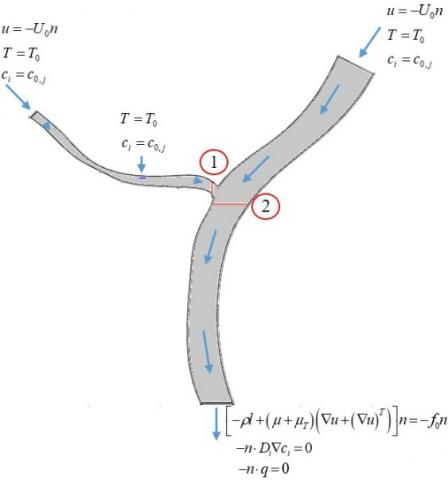(b) Equations of the boundary conditions

Figure 1. Geometry of confluent river model

This research investigates the velocity fields, pollutant concentration dispersions, and temperature distributions in two-dimensional model of the confluent river by analyzing the effects of the parameters. The boundary condition equations were illustrated in Figure 1(b). Moreover, the boundary conditions applied to the wall of the model are non-slip of fluid flow, non-heat flux and non-molar flux. Also, an initial temperature value of 27 °C and an initial pollutant concentration in the water of 0 mol.m-3 are applied to the surface of the model.

In the effects of the inlet flow velocities of the river, these velocities are 0.014, 0.035, and 0.056 m.s-1. These water velocities are the approximate water velocities in the Chao Phraya River, Thailand.

In the effects of the inlet flow velocities of the canal, these velocities are 0.0074, 0.011, and 0.015 m.s-1. These water velocities are modified to study the effects of them on the water velocity in the river. The inlet flow velocities of the canal of 0.011 and 0.015 m.s-1 are lower and higher than the inlet flow velocity of the river of 0.014 m.s-1, respectively.

Moreover, the water released at the inlet of both channels is considered that it is the pure water (temperature of 27 °C and pollutant concentration of 0 mol.m-3).

In the effects of the pollutant temperatures, these temperatures are 30, 50, and 70 °C. The temperature of wastewater from industry in Thailand is designated that should be less than 40 °C, which the pollutant temperatures of 30 and 50 °C are approximately different from it. Moreover, the pollutant temperature of 70 °C is much higher than the wastewater temperature.

2.2 Numerical procedures

The governing equations are solved numerically using the finite element method (FEM) via COMSOL MultiphysicsTM to demonstrate the velocity field, pollutant concentration dispersions, and temperature distributions .

## 2.jpg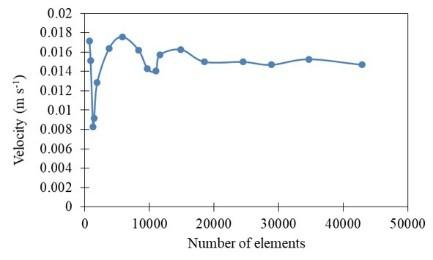Figure 2. Investigation of mesh independence

## 3.jpgFigure 3. Finite element mesh based on computational model

An investigation of mesh independence found 24,530 elements consisting of 19,783 triangular elements and 4,747 quadrilateral elements. The maximum element size is 16.3 m and, the minimum element size is 0.0485 m (Figures 2 and 3).

3. Validation

The pollutant concentration dispersion results are verified with the study results of Gualtieri by comparing the maximum pollutant concentration at each point in the x-axis. In this comparison, the results are similar for points near the pollutant source. For points away from the pollutant source, the results are different, which may be caused by the properties of substances and incomplete information of initial and boundary conditions. The comparison of the maximum pollutant concentration dispersion results is illustrated in Figure 4.

## 4.jpg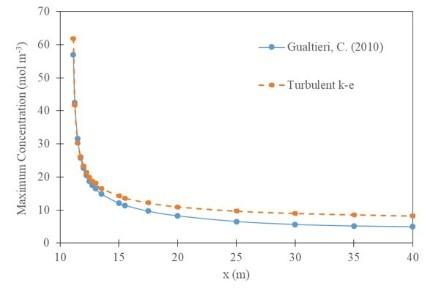Figure 4. Comparison of the maximum pollutant concentration dispersion results

4. Results

4.1 Velocity fields

The effects of the inlet flow velocities of both channels can affect the velocity fields in the confluent river. The water velocity of the river increases with the inlet flow velocities of both channel, especially with the effect of the inlet flow velocity of the river. A higher water velocity occurs near the left bank of the river. The water velocities of the river measured at the line 2 are illustrated in Figure 5.

## 5a.jpg(a) Effect of the inlet flow velocities of the canal

## 5b.jpg(b) Effect of the inlet flow velocities of the river

Figure 5. Water velocities of the river at the measured the line 2

## 6a.jpg(a) Effect of the inlet flow velocities of the canal

## 6b.jpg(b) Effect of the inlet flow velocities of the river

Figure 6. Water velocities of the canal measured at the line 1

The water velocity of the canal increases with the inlet flow velocity of the canal obviously. The water velocity near the top bank of the canal decreases with the inlet flow velocity of the river, whereas the water velocity near the bottom bank of the canal increases with the inlet flow velocity of the river. This is because the water of the river flows into the canal near the top bank of the canal. The water velocities of these phenomena are illustrated in Figure 6.

## 7a.jpg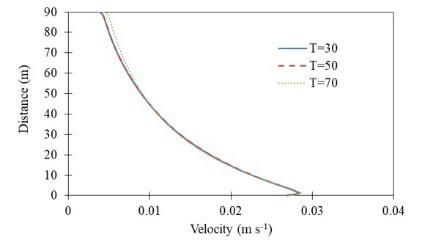(a) Water velocities of the canal measured at the line 1

## 7b.jpg(b) Water velocities of the river measured at the line 2

Figure 7. Water velocities affected by the pollutant temperatures with the inlet flow velocity of the canal of 0.011 m.s-1 and inlet flow velocity of the river of 0.056 m.s-1

## 8.jpg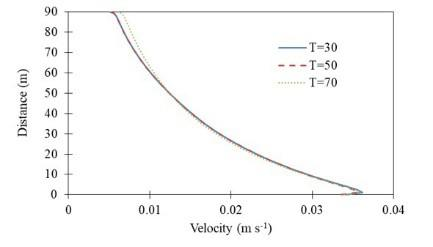Figure 8. Water velocities, measured at the line 1, affected by pollutant temperatures with the inlet flow velocity of the canal of 0.015 m.s-1 and inlet flow velocity of the river of 0.056 m.s-1

The pollutant temperatures slightly affect the water velocity of both channels (Figure 7). However, the pollutant temperature of 70 °C can increase the water velocity near the top bank of the canal due to higher temperature difference. It also increases the water velocity of the river. Moreover, the pollutant temperature can affect the water velocity with greater effect when the inlet flow velocities of both channels increase (Figure 8). However, the inlet flow velocities of both channels are the significant parameter which can affect the water velocity of both channels.

4.2 Pollutant concentration dispersions

The inlet flow velocities of both channel significantly influence the pollutant concentration dispersion. The water of the canal is contaminated by the pollutant. They flow together in the canal to the river. At a river confluence, the pollutant deposits due to a collision of the water. In the river, they are affected by the inlet flow velocities of both channels as mentioned in Section 4.1. In the first period, an average pollutant concentration ratio increases during the first 8 hours. Then, it begins to decrease because the pollutant emissions was stopped. In the general case, an increase in the average pollutant concentration ratio is greater at higher inlet flow velocity of the canal in the first period. This is because the higher inlet flow velocity of the canal can induce more pollutant from the pollutant source. However, it can send more pollutant into the river. Therefore, a decrease in the average pollutant concentration ratio is greater at higher inlet flow velocity of the canal in the last period. Moreover, the higher inlet flow velocity of the river can remove more pollutant. A quantity of the remaining pollutant in the river at the same time are lower at higher inlet flow velocity of the river (Figure 9). The average pollutant concentration ratios are illustrated in Figure 10. However, there are two cases that do not follow the general case mentioned above. These average pollutant concentration ratios varying with time are different from the general case in some period time.

## 9.jpg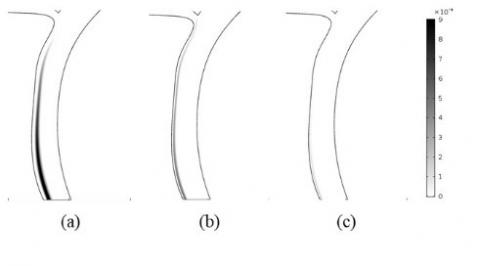Figure 9. Pollutant concentration dispersion affected by the inlet flow velocities of the river of (a) 0.014 m.s-1, (b) 0.035 m.s-1, and (c) 0.056 m.s-1

## 10a.jpg(a) Inlet flow velocity of the river of 0.035 m.s-1

## 10b.jpg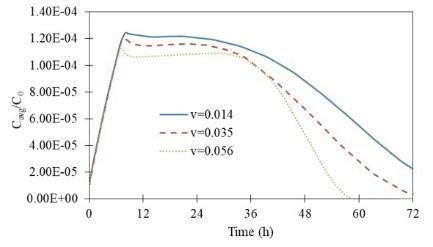(b) Inlet flow velocity of the canal of 0.011 m.s-1

Figure 10. Average pollutant concentration ratios

In the first different case, the inlet flow velocity of the canal is higher than the inlet flow velocity of the river, where the inlet flow velocity of the canal is 0.015 m.s-1, and the inlet flow velocity of the river is 0.014 m.s-1. This induces more pollutant in the canal to the river. Therefore, this can decrease the average pollutant concentration ratio, in the first period, to be lower than 0.00016 that diverges from the others (Figures 11 and 12).

## 11a.jpg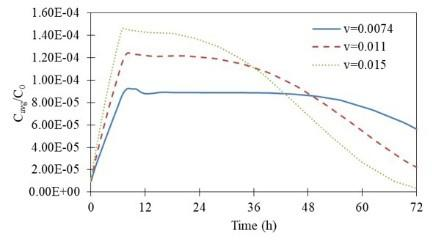(a) Effect of the inlet flow velocities of the canal

## 11b.jpg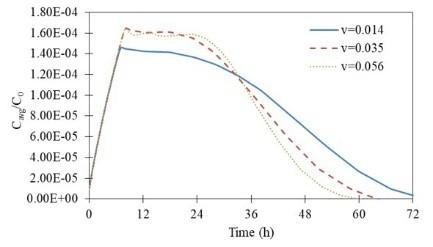(b) Effect of the inlet flow velocities of the river

Figure 11. Average pollutant concentration ratios (the inlet flow velocity of the canal is higher than the inlet flow velocity of the river)

## 12.jpg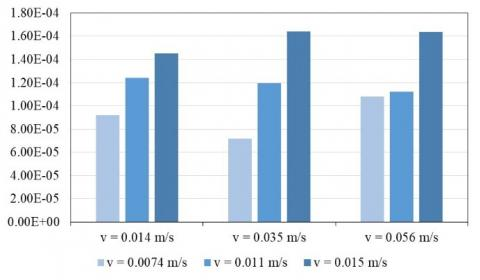Figure 12. Comparison of the maximum pollutant concentrations

## 13.jpg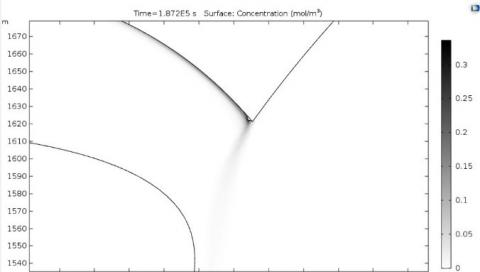Figure 13. Pollutant concentration deposition at the river confluence at 52 hours

In the last different case, the inlet flow velocity of the canal is too lower than the inlet flow velocity of the river, where the inlet flow velocity of the canal is 0.0074 m.s-1 and the inlet flow velocity of the river is 0.056 m.s-1. This is worse to induce the pollutant to the river as observed in the results of the water velocities in the canal at the measured line 1. The pollutant deposits at the river confluence (Figure 13). Therefore, the average pollutant concentration ratio increases when the pollutant arrives at the river confluence (Figure 14).

## 14a.jpg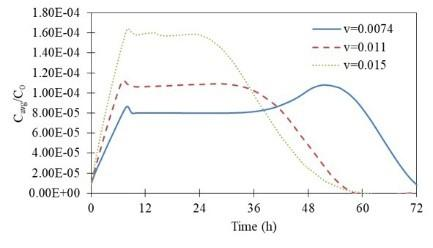(a) Average pollutant concentration ratios

## 14b.jpg(b) Maximum pollutant concentration ratios

Figure 14. Effects of the inlet flow velocity of the canal which is too lower than the inlet flow velocity of the river

## 15a.jpg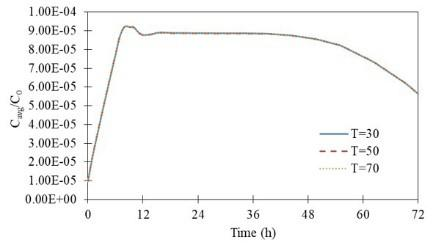(a) Inlet flow velocity of the river of 0.014 m.s-1

## 15b.jpg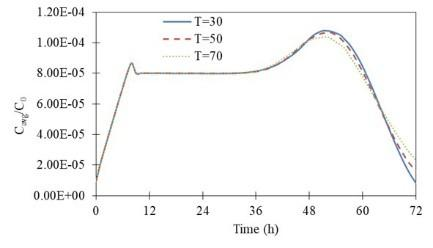(b) Inlet flow velocity of the river of 0.056 m.s-1

Figure 15. Effect of the pollutant temperature on the average pollutant concentration ratios

The pollutant temperatures slightly affect the pollutant concentration. Although, the pollutant dispersion are affected by the diffusion coefficients of the pollutants depended on the temperature, which mentioned in Section 2. Figure 15 shows a similar result of the average pollutant concentration ratio. This is because this river model is too large to be affected by the diffusion coefficient. Moreover, the pollutant temperature can affect the water velocity with greater effect when the inlet flow velocities of both channel are higher. Therefore, it indicates that the inlet flow velocities are mainly the parameters which can affect the pollutant concentration dispersion.

4.3 Temperature distributions

The highest maximum temperatures are equal to the pollutant temperatures. Fig. 16(b) shows that a decrease in the maximum temperature is greater at lower pollutant temperature. This is because the pollutant temperature increase associates with the pollutant increase. Moreover, Figure 16(a) and 16(c) also associate with the behavior described in section 4.2. The decrease in the maximum temperature is greater when the inlet flow velocity of the canal is higher than the inlet flow velocity of the river. Also, the decrease in the maximum temperature is lower when the inlet flow velocity of the canal is too lower than the inlet flow velocity of the river. The trend of the results still follows the results mentioned above, although the pollutant temperature is increased. The effects of the inlet flow velocities of both channels on the maximum temperatures are illustrated in Figures 16 and 17.

The variation in temperature distribution pattern conforms to the variation in pollutant concentration dispersion pattern (Figure 18). Also, the phenomena of the increase or decrease in the maximum temperatures are similar to the phenomena of the average pollutant concentration ratios as mentioned above.

## 16a.jpg(a) Inlet flow velocities of the river of 0.014 m.s-1

## 16b.jpg(b) Inlet flow velocities of the river of 0.035 m.s-1

## 16c.jpg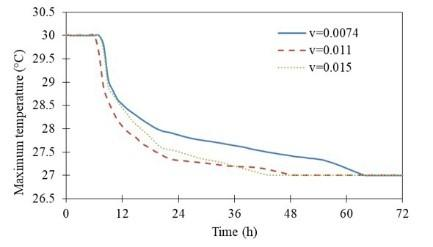(c) Inlet flow velocities of the river of 0.056 m.s-1

Figure 16. Maximum temperatures affected by the inlet flow velocities of the canal

## 17a.jpg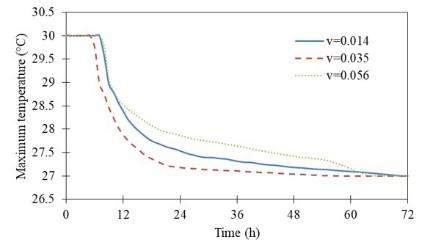(a) Inlet flow velocities of the canal of 0.0074 m.s-1

## 17b.jpg(b) Inlet flow velocities of the canal of 0.011 m.s-1

## 17c.jpg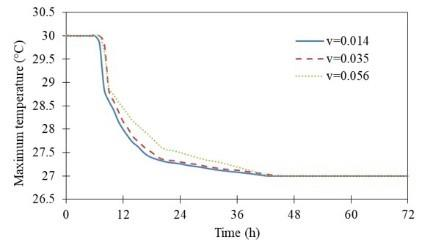(c) Inlet flow velocities of the canal of 0.015 m.s-1

Figure 17. Maximum temperatures affected by the inlet flow velocities of the river

## 18a.jpg(a) Pollutant concentration dispersion

## 18b.jpg(b) Temperature distribution

Figure 18. Comparison of the pollutant concentration dispersion and the temperature distribution

5. Conclusions

This article numerically studies the effects of the inlet flow velocity of the canal, the inlet flow velocity of the river and the pollutant temperatures in the two-dimensional confluent river model based on the finite element method.

The water velocity in the river increases with the inlet flow velocities of both channels, especially with the inlet flow velocity of the river. The water velocity in the canal increases with the inlet flow velocity of the canal. Besides, the inlet flow velocity of the river increases the water velocity at the bottom bank of the canal, but decreases it at the top bank of the canal. However, the pollutant temperature can slightly affect the water velocity.

In the first period, the increase in the pollutant concentration is greater at higher inlet flow velocity of the canal. However, the decrease in the pollutant concentration is also greater at   higher inlet flow velocities of both channels in the last period after stopping the pollutant emissions. Some cases are different from these phenomena. In the first different case, the inlet flow velocity of the canal is higher than the inlet flow velocity of the river. This makes its maximum pollutant concentration ratio decrease. In the last different case, the inlet flow velocity of the canal is too lower than the inlet flow velocity of the river. This makes the pollutant deposits at the river confluence, and its maximum pollutant concentration ratio increases higher than the others. The pollutant temperatures can slightly affect the pollutant concentration dispersions. Therefore, in this research, the higher inlet flow velocities of channels are suitable for removing the pollutants in the water, especially when the inlet flow velocity of the canal higher than it of the river. Moreover, the inlet flow velocity of the canal being too lower than the inlet flow velocity of the river should be avoided.

The temperature results conform to the pollutant concentration ratio. The trends of the maximum temperature are similar to the results of the effects of the inlet flow velocities of both channels on pollutant concentration. Although, the pollutant temperature is increased. The maximum temperature increases with the pollutant temperature. However, the pollutant temperature cannot affect the average temperature in the whole model.

The obtained results are beneficial for analysis of removing the pollutant out of the water source. The suitable water velocities at the inlets are the important factor for removing it. The pollutant temperature can slightly affect the water velocity and the pollutant concentration because the model is too large.

Acknowledgment

The Thailand Research Fund (Contract No. RTA 5980009) and The Thailand Government Budget Grant provided financial support for this study.

Nomenclature
 A heat transfer area of surface, m2 cp specific heat, J. kg-1. K-1 C D pollutant concentration, mol.m-3 diffusion coefficient, m2.s-1 h convective heat transfer coefficient, W.m-2.K-1 k thermal conductivity, W.m-1.K-1 p pressure, Pa S pollutant concentration at the pollutant source, mol.m-3.s-1 T temperature, °C u velocity of fluid in x-axis, m.s-1 v velocity of fluid in y-axis, m.s-1 Greek symbols r density of fluid, kg.m-3 Subscripts avg average max maximum 0 Initial value of boundary condition
References

 Owa FD. (2013). Water pollution: Sources, effects, control and management. Mediterranean Journal of Social Sciences 4(8): 65–68. https://doi.org/10.5901/mjss.2013.v4n8p65

 Neighborhood, Water Quality: Pollution and Water Quality. (2000). Project Oceanography, Tampa, USA.

 Caissie D, Satish MG, El-Jabi N. (2007). Predicting water temperatures using a deterministic model: Application on Miramichi River Catchments (New Brunswick, Canada). Journal of Hydrology 336(3–4): 303-315. https://doi.org/10.1016/j.jhydrol.2007.01.008

 Ministry of Natural Resources and Environment, PCD, Thailand. (2016). PCD: Water Quality Stansards.http://www.pcd.go.th/info_serv/reg_std_water04.html.

 Pimpunchat B, Sweatman WL, Wake GC, Triampo W, Parshotam A. (2009). A mathematical model for pollution in a river and its remediation by aeration. Applied Mathematics Letters 22 (3): 304-308. https://doi.org/10.1016/j.aml.2008.03.026

 Park I, Seo IW. (2018). Modeling non-Fickian pollutant mixing in open channel flows using two-dimensional particle dispersion model. Advances in Water Resources 111: 105–120. https://doi.org/10.1016/j.advwatres.2017.10.035

 Shams M, Ahmadi G, Smith DH. (2002). Computational modeling of flow and sediment transport and deposition in meandering rivers. Advances in water resources 25(6): 689–699. https://doi.org/10.1016/S0309-1708(02)00034-9

 Li X, Wang YG, Zhang SX. (2009). Numerical simulation of water quality in Yangtze Estuary. Water Science and Engineering 2(4): 40–51. https://doi.org/10.3882/j.issn.1674-2370.2009.04.004

 Gualtieri C. (2010). RANS-based simulation of transverse turbulent mixing in a 2D geometry. Environmental Fluid Mechanics 10(1–2): 137–156. https://doi.org/10.1007/s10652-009-9119-6

 Biron PM, Ramamurthy AS, Han S. (2004). Three-dimensional numerical modeling of mixing at river confluences. Journal of Hydraulic Engineering 130(3): 243–253. https://doi.org/10.1061/(ASCE)0733-9429(2004)130:3(243)

 Bradbrook KF, Biron PM, Lane SN, Richards KS, Roy AG. (1998). Investigation of controls on secondary circulation in a simple confluence geometry using a three‐dimensional numerical model. Hydrological Processes 12(8): 1371–1396. https://doi.org/10.1002/(SICI)1099-1085(19980630)12:8<1371::AID-HYP620>3.0.CO;2-C

 Bradbrook KF, Lane SN, Richards KS, Biron PM, Roy AG. (2001). Role of bed discordance at asymmetrical river confluences. Journal of Hydraulic Engineering 127(5): 351–368. https://doi.org/10.1061/(ASCE)0733-9429(2001)127:5(351)

 Radu VM, Diacu E, Moncea MA, Dumitru FD, Panait AM, Ionescu P. (2017). Numerical modelling of pollutant dispersion in the Lower Danube River. Revista de Chimie 68(11): 2477–2481.

 Tsydenov BO, Kay A, Starchenko AV. (2015). Numerical modelling of pollutant propagation in Lake Baikal during the spring thermal bar. Procedia Computer Science 51: 2658–2662. https://doi.org/10.1016/j.procs.2015.05.373

 Lopes LFG, Do Carmo JSA, Cortes RMV, Oliveira D. (2004). Hydrodynamics and water quality modelling in a regulated river segment: Application on the instream flow definition. Ecological Modelling 173(2-3): 197-218. https://doi.org/10.1016/j.ecolmodel.2003.07.009

 Antonopoulos VZ,Gianniou SK. (2003). Simulation of water temperature and dissolved oxygen distribution in Lake Vegoritis, Greece. Ecological Modelling 160(1-2): 39-53. https://doi.org/10.1016/S0304-3800(02)00286-7

 Jin G, Tang H, Li L, Barry DA. (2015). Prolonged river water pollution due to variable‐density flow and solute transport in the riverbed. Water Resources Research 51(4): 1898–1915. https://doi.org/10.1002/2014WR016369

 Tantemsapya N. (2017). Modeling approach to water quality management in the lower Pong river, Thailand. Asia-Pacific Journal of Science and Technology 13 (10): 1199-1206.

 Cristea VM, Bâgiu ED, Agachi PŞ. (2010). Simulation and control of pollutant propagation in Someş river using COMSOL multiphysics. Computer Aided Chemical Engineering 28: 985–990. https://doi.org/10.1016/S1570-7946(10)28165-8

 Cristea VM. (2013). Counteracting the accidental pollutant propagation in a section of the River Someş by automatic control. Journal of Environmental Management 128: 828–836. https://doi.org/10.1016/j.jenvman.2013.06.016

 Petrescu V, Sumbasacu O. (2010). Comparison between numerical simulation and measurements of the pollutant dispersion in a river. Case study. UPB Sci. Bull., Series D 72(3): 157–164.

 Saltzman E, King D, Holmen K, Leck C. (1993). Experimental determination of the diffusion coefficient of dimethylsulfide in water. Journal of Geophysical Research: Oceans 98(C9): 16481–16486.

 COMSOL Multiphysics™ Version 4.4: User’s Guide and Reference Manual. (2014). Comsol, Burlington, MA, USA.